### Seeing is believing!

Before you order, simply sign up for a free user account and in seconds you'll be experiencing the best in CFA exam preparation.

##### Subject 8. Interest Rate Swap Contracts
To understand swaps, let us look at an example.

On December 15 XYZ enters into a \$50 million NP swap with ABSwaps. Payments will be on the 15th of March, June, September, and December for one year, based on LIBOR. XYZ will pay 7.5% fixed and ABSwaps will pay LIBOR. Interest is based on exact day counts and 360 days (30 per month).

In general, the cash flow to the fixed payer will be:

Notional principal x (LIBOR - Fixed rate) x (Days / 360 or 365)

• The payments in this swap are: 50,000,000 x (LIBOR - 0.075) x (days/360). Note that payments are netted.
• We denote Li(m) as the m-day LIBOR on day i. If i = 1, we are referring to 90 days after day 0 in this example, and L1(90) is the 90-day LIBOR on day 90.
• q represents the factor (days/360).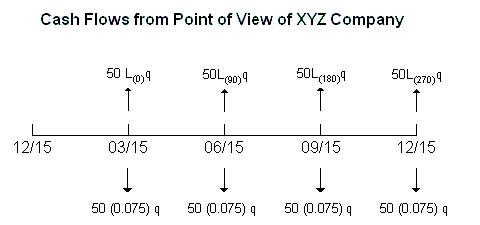After-the-fact payments: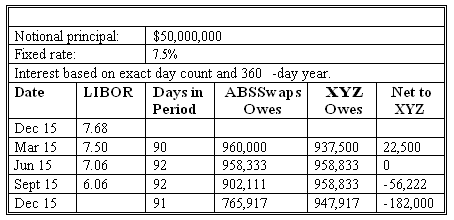Note: This combination of LIBORS on the above dates represents only one of an infinite number of possible outcomes to the swap. They are used to illustrate how the payments are determined and not the likely results.

Pricing an Interest Rate Swap

Remember that swap pricing means determining the fixed rate at the start of the swap.

We denote PV0,ti(1) as the present value factor on a zero-coupon instrument paying \$1 at its maturity date. The price of a LIBOR zero coupon bond with a maturity of ti days is 1/{1 + L0(ti) * (ti/360)}. As an example, to discount payments 180 days later, we multiply the payment amount by the following factor: PV0, 180(1) = 1 / {1 + [L0(180) x (180/360)]}. We can think of this discount factor as the value of a spot LIBOR deposit that pays \$1 after 180 days.

On day 0, the floating rate is set for the first period and the interest is to be paid at that rate on day 90. Then, on day 90, the rate is set for the second period and the interest is paid on day 180. This process continues so that on day 270 the rate is set for the last period, and the final interest payment and the principal are paid on day 360. Let the principal be 1.0.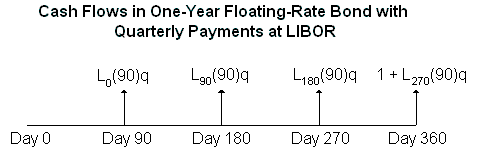Starting at the maturity date and working back, we can see that the value is par at the start and on each coupon reset date.

• Suppose today is day 270, and the LIBOR today is L270(90). Remember that this rate is the 90-day LIBOR in the market at the time. Looking ahead to day 360, we anticipate receiving 1.0, the final principal payment, plus L270(90) x 90/360. What is the value of this amount on day 270? We would discount it by the appropriate 90-day LIBOR in the following manner:

Value on day 270 = (Payment on day 360) x (One-period discount factor) = [1.0 + L270(90) x (90/360)] x {1 / [1 + (L270(90) x (90/360))]} = 1.0

• We continue this procedure, stepping back until we reach time 0.

Payments in an interest rate swap do not include an initial or final exchange of notional principals. If we introduce the notional principal payments as though they were actually made, we have not done any harm. The cash flows on the swap are still the same. However, by adding the notional principals, we can separate the cash flow streams of an interest rate swap into those of a fixed-rate bond and a floating-rate bond.

The value of a swap is: V = value of fixed bond (FB) - Value of floating bond (VB).

• The value of floating bond: VB = 1 (at time 0 or a payment date).
• The value of fixed bond is simply the sum of the present value factors for each payment.

To price the swap at the start, set V to zero.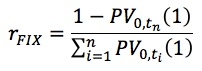The expression PV0, tn(1) is the present value factor for the final hypothetical notional principal payment of 1.

In the above example, suppose the term structure of LIBOR at the start of the contract is: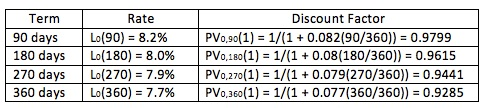The annualized fixed rate would, therefore, be R = (360/90) [(1 - 0.9285) / (0.9799 + 0.9615 + 0.9441 + 0.9285)] = 0.075.

Thus, the rate would be 7.5%.

Valuing an Interest Rate Swap

The value of a fixed rate swap at some future point in time, t, is simply the sum of the present value of the difference in fixed swap rates multiplied by the stated notional amount (denoted NA), or:where:

• FS0 is the fixed rate established at the start of the swap.
• FSt is the fixed rate established at Time t.

User Comment
shasha for year 5, B took rate of 7.5% + 1% for its payment. LIBOR 7.75% is no use in this situation.
haarlemmer Typically, it is set in advance. It may be adjusted to reflect the current rate though.
Kashi2010 haarlemmer - no, sorry.

Swap contracts typically define a rate reset date as something like '20th of March, June, September, & December' (for quarterly floaters), and will specify a setting date to reference. For example T-2 will look at the Libor (e.g.) set two business days before the reset date - in this example that would be the 18th (assuming no weekends/holidays in between).

They pay no attention to movements thereafter during the period - so for the full quarter payments will calculate off the initial rate set.
rascallian any idea why in the denominator for the price of the bond, the (1+Lo(t)) is multiplied by t/360 rather than being to the power of? i.e. (1+Lo(t)^(t/360))
johntan1979 rascallian must have studied too hard to be seeing imaginary stuff in the notes
bidisha Lol
birdperson now that i learned this, it better be on that test...